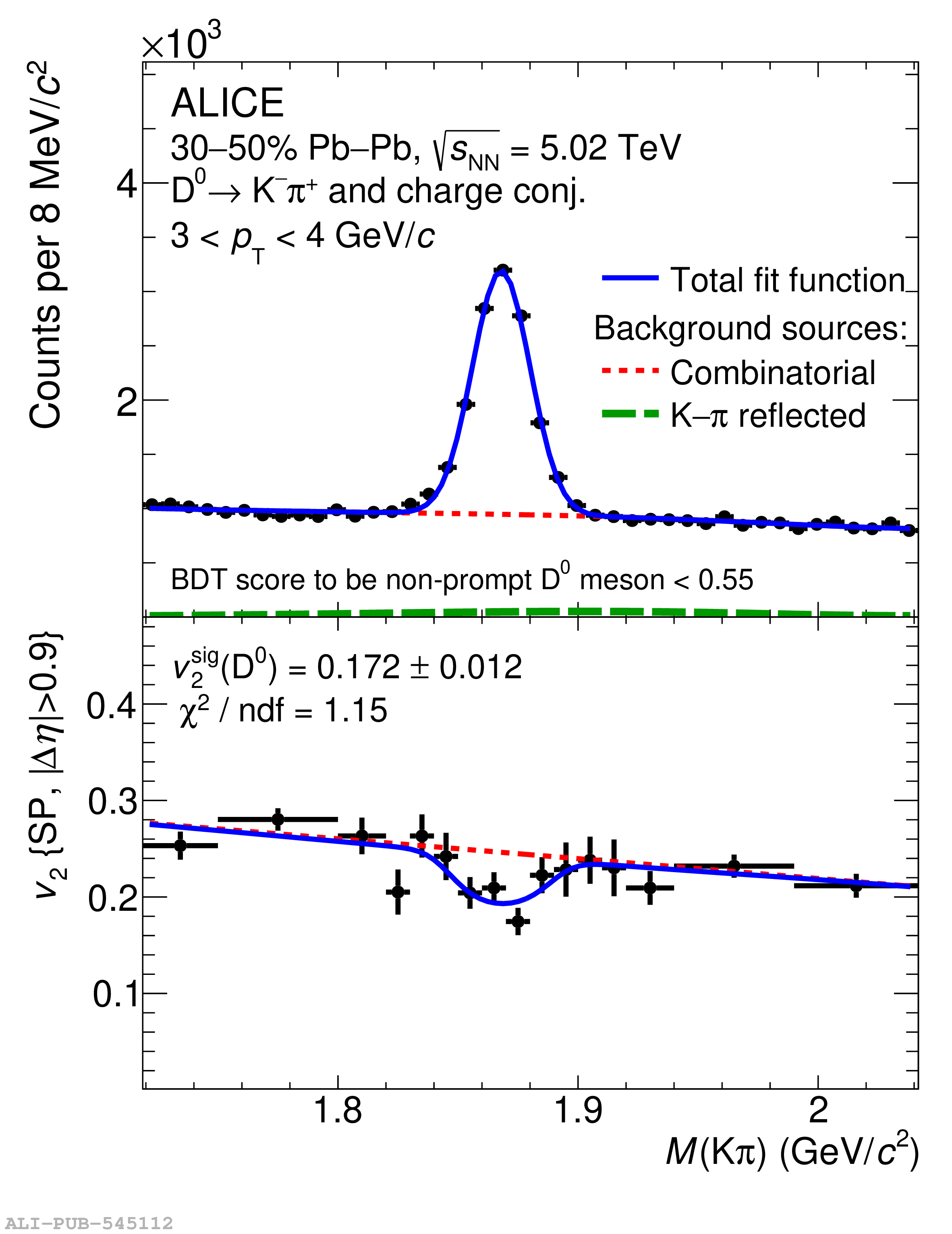# Figure 1

 Simultaneous fits of the invariant-mass distribution and $v_2^\mathrm{tot}(M_\mathrm{K\pi})$ of $\Dzero$ mesons in $3 < \pt < 4 \GeV/c$. Left panel: fits using $\Dzero$-meson candidates with low probability to be a non-prompt \Dzero meson. Right panel: fits using $\Dzero$-meson candidates with high probability to be a non-prompt \Dzero meson. The corresponding BDT score selection for the measured raw yield is reported. The blue lines, the dotted red curves, and the green solid lines represent the total fit function, the combinatorial-background fit function, and the contribution of the reflected signal, respectively.